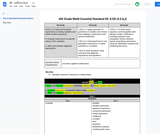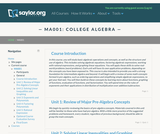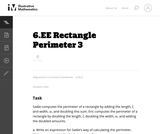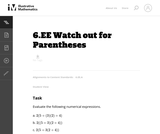Updating search results...

# 12 Results

View
Selected filters:
• MCCRS.Math.Content.6.EE.A.2bConditional Remix & Share Permitted
CC BY-NC-SA
Rating
0.0 stars

Create and evaluate expressions involving variables and whole number exponents.

b. Evaluate expressions at specific values of the variables.

d. Write and evaluate algebraic expressions.

Subject:
Mathematics
Material Type:
Activity/Lab
Author:
Liberty Public Schools
Date Added:
04/12/2021Unrestricted Use
CC BY
Rating
4.0 stars

This course is also intended to provide the student with a strong foundation for intermediate algebra and beyond. Upon successful completion of this course, you will be able to: simplify and solve linear equations and expressions including problems with absolute values and applications; solve linear inequalities; find equations of lines; and solve application problems; add, subtract, multiply, and divide various types of polynomials; factor polynomials, and simplify square roots; evaluate, simplify, multiply, divide, add, and subtract rational expressions, and solve basic applications of rational expressions. This free course may be completed online at any time. It has been developed through a partnership with the Washington State Board for Community and Technical Colleges; the Saylor Foundation has modified some WSBCTC materials. (Mathematics 001)

Subject:
Algebra
Material Type:
Full Course
Provider:
The Saylor Foundation
Date Added:
04/16/2012Read the Fine Print
Educational Use
Rating
0.0 stars

The CyberSquad tries to figure out how Hackerë_í__ cyberfrog moves when its various buttons are pressed, in this video from Cyberchase.

Subject:
Mathematics
Algebra
Material Type:
Lecture
Provider:
PBS LearningMedia
Provider Set:
PBS Learning Media: Multimedia Resources for the Classroom and Professional Development
Author:
U.S. Department of Education
WNET
Date Added:
07/11/2008Read the Fine Print
Educational Use
Rating
0.0 stars

In this video from Cyberchase, the CyberSquad must figure out the new input/output pattern on Hackerë_í__ larger cyberfrog.

Subject:
Mathematics
Algebra
Material Type:
Lecture
Provider:
PBS LearningMedia
Provider Set:
PBS Learning Media: Multimedia Resources for the Classroom and Professional Development
Author:
U.S. Department of Education
WNET
Date Added:
07/11/2008Only Sharing Permitted
CC BY-NC-ND
Rating
4.0 stars

This lesson unit is intended to help you assess how well students are able to: Perform arithmetic operations, including those involving whole-number exponents, recognizing and applying the conventional order of operations; Write and evaluate numerical expressions from diagrammatic representations and be able to identify equivalent expressions; apply the distributive and commutative properties appropriately; and use the method for finding areas of compound rectangles.

Subject:
Geometry
Material Type:
Assessment
Lesson Plan
Provider:
Shell Center for Mathematical Education
Provider Set:
Mathematics Assessment Project (MAP)
Date Added:
04/26/2013Conditional Remix & Share Permitted
CC BY-NC
Rating
0.0 stars

Subject:
Mathematics
Material Type:
Full Course
Provider:
Pearson
Date Added:
10/06/2016Rating
0.0 stars

Expressions

Type of Unit: Concept

Prior Knowledge

Students should be able to:

Write and evaluate simple expressions that record calculations with numbers.
Use parentheses, brackets, or braces in numerical expressions and evaluate expressions with these symbols.
Interpret numerical expressions without evaluating them.

Lesson Flow

Students learn to write and evaluate numerical expressions involving the four basic arithmetic operations and whole-number exponents. In specific contexts, they create and interpret numerical expressions and evaluate them. Then students move on to algebraic expressions, in which letters stand for numbers. In specific contexts, students simplify algebraic expressions and evaluate them for given values of the variables. Students learn about and use the vocabulary of algebraic expressions. Then they identify equivalent expressions and apply properties of operations, such as the distributive property, to generate equivalent expressions. Finally, students use geometric models to explore greatest common factors and least common multiples.

Subject:
Mathematics
Algebra
Material Type:
Unit of Study
Provider:
PearsonConditional Remix & Share Permitted
CC BY-NC
Rating
0.0 stars

Students play an Expressions Game in which they describe expressions to their partners using the vocabulary of expressions: term, coefficient, exponent, constant, and variable. Their partners try to write the correct expressions based on the descriptions.Key ConceptsMathematical expressions have parts, and these parts have names. These names allow us to communicate with others in a precise way.A variable is a symbol (usually a letter) in an expression that can be replaced by a number.A term is a number, a variable, or a product of numbers and variables. Terms are separated by the operator symbols + (plus) and – (minus).A coefficient is a symbol (usually a number) that multiplies the variable in an algebraic expression.An exponent tells how many copies of a number or variable are multiplied together.A constant is a number. In an expression, it can be a constant term or a constant coefficient. In the expression 2x + 3, 2 is a constant coefficient and 3 is a constant term.Goals and Learning ObjectivesIdentify parts of an expression using appropriate mathematical vocabulary.Write expressions that fit specific descriptions (for example, the expression is the sum of two terms each with a different variable).

Subject:
Algebra
Material Type:
Lesson Plan
Provider:
Pearson
Date Added:
09/21/2015Conditional Remix & Share Permitted
CC BY-NC
Rating
0.0 stars

Students are introduced to classroom routines and expectations, and complete a full mathematics lesson. The class discusses how to clearly present work to classmates. Partner work is modeled, and partners then work to match numerical expressions to corresponding word descriptions. Students read and discuss a summary of the math in the lesson, and then write a reflection about their thoughts.Key ConceptsStudents match a numerical expression to its corresponding description in words. Students interpret parentheses and brackets in numerical expressions and they construct viable arguments and critique the reasoning of others. Students learn to use the exponent 2 to represent squaring.Goals and Learning ObjectivesDescribe the classroom routines and expectations.Consider how to present work clearly to classmates.Collaborate with a partner.Critique a partner’s reasoning.Connect a numerical expression to its corresponding word description.Learn to use an exponent of 2 to represent squaring.

Subject:
Algebra
Material Type:
Lesson Plan
Provider:
Pearson
Date Added:
09/21/2015
RemixConditional Remix & Share Permitted
CC BY-NC-SA
Rating
0.0 stars

Students are introduced to classroom routines and expectations, and complete a full mathematics lesson. The class discusses how to clearly present work to classmates. Partner work is modeled, and partners then work to match numerical expressions to corresponding word descriptions. Students read and discuss a summary of the math in the lesson, and then write a reflection about their thoughts.Key ConceptsStudents match a numerical expression to its corresponding description in words. Students interpret parentheses and brackets in numerical expressions and they construct viable arguments and critique the reasoning of others. Students learn to use the exponent 2 to represent squaring.Goals and Learning ObjectivesDescribe the classroom routines and expectations.Consider how to present work clearly to classmates.Collaborate with a partner.Critique a partner’s reasoning.Connect a numerical expression to its corresponding word description.Learn to use an exponent of 2 to represent squaring.

Subject:
Algebra
Material Type:
Lesson Plan
Provider:
Pearson
Author:
Benjamin White
Date Added:
08/23/2018Unrestricted Use
CC BY
Rating
0.0 stars

The purpose of this task is to ask students to write expressions and to consider what it means for two expressions to be equivalent.

Subject:
Mathematics
Material Type:
Activity/Lab
Provider:
Illustrative Mathematics
Provider Set:
Illustrative Mathematics
Author:
Illustrative Mathematics
Date Added:
08/07/2012Unrestricted Use
CC BY
Rating
0.0 stars

This problem asks the student to evaluate three numerical expressions that contain the same integers yet have differing results due to placement of parentheses.

Subject:
Mathematics
Material Type:
Activity/Lab
Provider:
Illustrative Mathematics
Provider Set:
Illustrative Mathematics
Author:
Illustrative Mathematics
Date Added:
08/27/2012